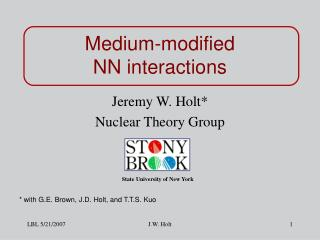DownloadDownload PresentationMedium-modified NN interactions

Medium-modified NN interactions

Download PresentationMedium-modified NN interactions

- - - - - - - - - - - - - - - - - - - - - - - - - - - E N D - - - - - - - - - - - - - - - - - - - - - - - - - - -
Presentation Transcript

1. Medium-modified NN interactions Jeremy W. Holt* Nuclear Theory Group State University of New York * with G.E. Brown, J.D. Holt, and T.T.S. Kuo J.W. Holt

2. Medium-modified Microscopic Foundation for density-dependent interactions Can such interactions provide a good description of nuclear systems? J.W. Holt

3. Chiral Effective Theories (e.g. Hidden Local Symmetry) (1) Finite nuclei (2) Nuclear matter (3) Hot/dense matter Double Decimation Describe in a unified way G. Brown and M. Rho, Phys. Rept. 396 (2004) Chiral decimation QCD Match T and  dependence with QCD Sum Rule correlation functions Fermi liquid decimation EFT with intrinsic T and  dependence Low-momentum interaction Traditional OBE potentials (e.g. Bonn, Nijmegen, etc.) Introduce medium modifications “by hand” J.W. Holt

4. In-medium modifications (T = 0,  0) Change in mass at  = 0TheoryExperimentUs ,  ~ 10 – 15% decrease Yes Yes 15%  ~ small increase Yes Yes 0%  ~ 10% decrease Yes No 7% Assume linear scaling: J.W. Holt

5. Traditional problem: Strong short distance repulsion (a) G-matrix RG and EFT (b) Vlow-k J.W. Holt

6. Bare NN Potentials Universal interaction for  = 2.1 fm-1 k = 2.1 fm-1  Elab = 350 MeV Integrate out the experimentallyunconstrained part of the NN interaction J.W. Holt

7. Outline of Results • Nuclear matter • Easier than finite nuclei (only one density) • Saturation (E/A, kF, K ) • Hartree-Fock (preliminary) • Ring diagram expansion (preliminary) • Fermi liquid theory [JWH et al., NPA 785 (2007) 322.] • Finite nuclei • Diminishing tensor force • Beta decay of 14C J.W. Holt

8. Saturation with low momentum interactions Fixed cutoff in Hartree-Fock approximation (no saturation) Empirical saturation energy and density Add leading order chiral 3N force S. Bogner et al., NPA 763 (2005) 59. J.W. Holt

9. Tensor force with dropping masses J.W. Holt

10. Hartree-Fock + Preliminary! Novel saturation mechanism J.W. Holt

11. Ring diagrams (pp) + + + Introduce model space m ~ 3.0 fm –1 Choose vlowk = m J.W. Holt

12. Preliminary! [MeV] [fm–1] J.W. Holt

13. Fermi Liquid Theory Weakly interacting quasiparticles Strongly interacting, normalFermi systems at T = 0 Quasiparticles defined only near Fermi surface:k kF Expand: quasiparticle interaction Hartree-Fock J.W. Holt

14. Spin & Isospin Dependence: Dimensionless parameters: Correspondence between FLP and observables J.W. Holt

15. Babu-Brown induced interaction S. Babu and G. Brown, Ann. Phys. 78 (1973) 1 + Pauli Principle  Sum Rules: J.W. Holt

16. Driving Terms (Vlow-k CD-Bonn) Full Calculation: Fd0= -1.20 Fd1= -0.50 Gd0= 0.14 Gd1= 0.24 F'd0= 0.35 F'd1= 0.26 G'd0= 0.60 G'd1= 0.12 Unstable (negative K) Rapid convergence of iteration scheme Sum Rules: Largest effect is to cut down the strong attraction in F channel J.W. Holt

17. How to improve? 1. Explicit three-body forces 2. In-medium modifications to NN interaction Extend Walecka mean field theory to constituent quarks Medium modifications equivalent to a type of 3N force J.W. Holt

18. Full many-body calculations gA = 1.25 gA* = 1.00 Decreasing g2wNN by 20% cuts K by 50% but changes other observables by ~ 5% quark model J.W. Holt

19. Finite nuclei Decreasing tensor force necessary! • Decay of 14C • 0– T=1 and 0– T=0 splitting in 16O • E2 and M1 moments of 6Li Traditional many-body effects or novel scaling mechanism? J.W. Holt

20. J.W. Holt

21. 14C 0+ 2 holes in 0p-shell – 1+ 14N Nijmegen I Preliminary J.W. Holt

22. Summary • NN interactions with medium-modified mesons • Saturation of nuclear matter and Fermi liquid parameters improved with dropping masses Outlook • Full analysis of ring diagrams • Look for nuclear observables where tensor force plays dominant role • Understand the connection between medium modifications and three-body forces J.W. Holt